# \$B%=!<%[!

C/2002 R4 = C/2007 Y4 ( SOHO )###\$B%^!<%9%G%s72\$N7OE}?^(B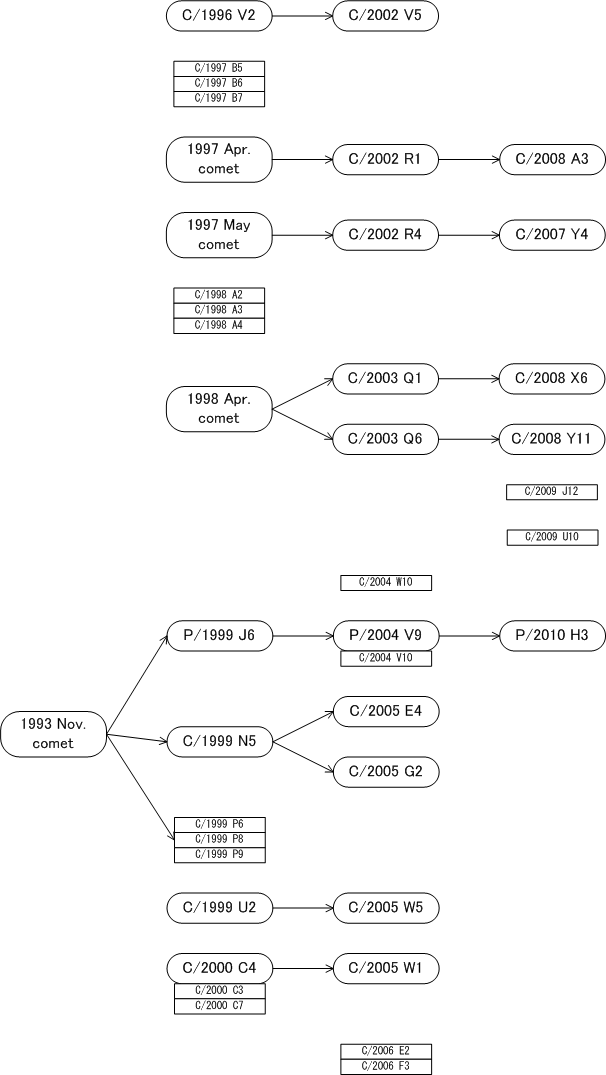\$B@8\$-;D\$C\$F\$\$\$kWB@1(B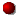\$B>CLG\$7\$?WB@1(BC/1996 V2 = C/2002 V5C/1997 B5C/2002 R1 = C/2008 A3C/2002 R4 = C/2007 Y4C/1998 A2C/2003 Q1 = C/2008 X6C/2003 Q6 = C/2008 Y11C/2009 J12C/2009 U10C/2004 W10P/1999 J6 = P/2004 V9 = P/2010 H3C/1999 N5 = C/2005 E4C/1999 N5 = C/2005 G2C/1999 P6C/1999 U2 = C/2005 W5C/2000 C4 = C/2005 W1C/2006 E2

###\$B2s5"5-O?(B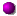\$BH/8+(B\$B=P8=(B\$B4QB,\$5\$l\$J\$+\$C\$?2s5"(B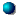\$BH/8+A0\$N=P8=(B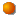\$BH/8+A0\$N4QB,\$5\$l\$J\$+\$C\$?2s5"(B\$B>-Mh\$N2s5"(BC/2007 Y4 2007/12/22C/2002 R4 2002/09/031997/05/12##### C/2002 R4 \$B\$H(B C/2007 Y4 \$B\$NF1Dj\$O(BM.P.E.C. 2008-B49\$B\$K7G:\\$5\$l\$?\$b\$N!#(B 1997\$BG/\$N6aF|E@DL2aF|\$O(BM.P.E.C. 2008-B49\$B\$K7G:\\$5\$l\$?\$b\$N!#(B